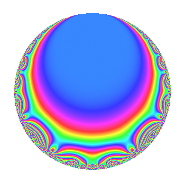# Properties

 Label 6027.2.a.qLevel 6027 Weight 2 Character orbit 6027.a Self dual Yes Analytic conductor 48.126 Analytic rank 0 Dimension 3 CM No Inner twists 1

# Related objects

## Newspace parameters

 Level: $$N$$ = $$6027 = 3 \cdot 7^{2} \cdot 41$$ Weight: $$k$$ = $$2$$ Character orbit: $$[\chi]$$ = 6027.a (trivial)

## Newform invariants

 Self dual: Yes Analytic conductor: $$48.1258372982$$ Analytic rank: $$0$$ Dimension: $$3$$ Coefficient field: 3.3.1620.1 Coefficient ring: $$\Z[a_1, \ldots, a_{5}]$$ Coefficient ring index: $$1$$ Fricke sign: $$-1$$ Sato-Tate group: $\mathrm{SU}(2)$

## $q$-expansion

Coefficients of the $$q$$-expansion are expressed in terms of a basis $$1,\beta_1,\beta_2$$ for the coefficient ring described below. We also show the integral $$q$$-expansion of the trace form.

 $$f(q)$$ $$=$$ $$q + q^{3} -2 q^{4} + \beta_{1} q^{5} + q^{9} +O(q^{10})$$ $$q + q^{3} -2 q^{4} + \beta_{1} q^{5} + q^{9} + ( -\beta_{1} + \beta_{2} ) q^{11} -2 q^{12} + ( 1 + \beta_{1} + \beta_{2} ) q^{13} + \beta_{1} q^{15} + 4 q^{16} + 2 q^{17} + ( 3 + \beta_{1} ) q^{19} -2 \beta_{1} q^{20} + ( 2 + \beta_{1} ) q^{23} + ( 3 + 2 \beta_{1} + \beta_{2} ) q^{25} + q^{27} + 2 \beta_{1} q^{29} + ( -1 - 2 \beta_{1} + \beta_{2} ) q^{31} + ( -\beta_{1} + \beta_{2} ) q^{33} -2 q^{36} - q^{37} + ( 1 + \beta_{1} + \beta_{2} ) q^{39} + q^{41} + ( -7 - \beta_{2} ) q^{43} + ( 2 \beta_{1} - 2 \beta_{2} ) q^{44} + \beta_{1} q^{45} + ( \beta_{1} + 3 \beta_{2} ) q^{47} + 4 q^{48} + 2 q^{51} + ( -2 - 2 \beta_{1} - 2 \beta_{2} ) q^{52} + ( -2 - 2 \beta_{1} - 2 \beta_{2} ) q^{53} + ( -10 - 2 \beta_{1} - 3 \beta_{2} ) q^{55} + ( 3 + \beta_{1} ) q^{57} + ( -6 - 2 \beta_{1} - 2 \beta_{2} ) q^{59} -2 \beta_{1} q^{60} + ( -2 - 2 \beta_{1} - 3 \beta_{2} ) q^{61} -8 q^{64} + ( 6 + 3 \beta_{1} - \beta_{2} ) q^{65} + ( 1 - \beta_{1} - \beta_{2} ) q^{67} -4 q^{68} + ( 2 + \beta_{1} ) q^{69} + ( 2 \beta_{1} + 2 \beta_{2} ) q^{71} + ( 7 + \beta_{2} ) q^{73} + ( 3 + 2 \beta_{1} + \beta_{2} ) q^{75} + ( -6 - 2 \beta_{1} ) q^{76} + ( 7 - \beta_{1} + 2 \beta_{2} ) q^{79} + 4 \beta_{1} q^{80} + q^{81} + ( 2 + \beta_{1} + 4 \beta_{2} ) q^{83} + 2 \beta_{1} q^{85} + 2 \beta_{1} q^{87} + ( -4 - \beta_{1} - 3 \beta_{2} ) q^{89} + ( -4 - 2 \beta_{1} ) q^{92} + ( -1 - 2 \beta_{1} + \beta_{2} ) q^{93} + ( 8 + 5 \beta_{1} + \beta_{2} ) q^{95} + ( -6 + 4 \beta_{1} ) q^{97} + ( -\beta_{1} + \beta_{2} ) q^{99} +O(q^{100})$$ $$\operatorname{Tr}(f)(q)$$ $$=$$ $$3q + 3q^{3} - 6q^{4} + 3q^{9} + O(q^{10})$$ $$3q + 3q^{3} - 6q^{4} + 3q^{9} - 6q^{12} + 3q^{13} + 12q^{16} + 6q^{17} + 9q^{19} + 6q^{23} + 9q^{25} + 3q^{27} - 3q^{31} - 6q^{36} - 3q^{37} + 3q^{39} + 3q^{41} - 21q^{43} + 12q^{48} + 6q^{51} - 6q^{52} - 6q^{53} - 30q^{55} + 9q^{57} - 18q^{59} - 6q^{61} - 24q^{64} + 18q^{65} + 3q^{67} - 12q^{68} + 6q^{69} + 21q^{73} + 9q^{75} - 18q^{76} + 21q^{79} + 3q^{81} + 6q^{83} - 12q^{89} - 12q^{92} - 3q^{93} + 24q^{95} - 18q^{97} + O(q^{100})$$

Basis of coefficient ring in terms of a root $$\nu$$ of $$x^{3} - 12 x - 14$$:

 $$\beta_{0}$$ $$=$$ $$1$$ $$\beta_{1}$$ $$=$$ $$\nu$$ $$\beta_{2}$$ $$=$$ $$\nu^{2} - 2 \nu - 8$$
 $$1$$ $$=$$ $$\beta_0$$ $$\nu$$ $$=$$ $$\beta_{1}$$ $$\nu^{2}$$ $$=$$ $$\beta_{2} + 2 \beta_{1} + 8$$

## Embeddings

For each embedding $$\iota_m$$ of the coefficient field, the values $$\iota_m(a_n)$$ are shown below.

For more information on an embedded modular form you can click on its label.

Label $$\iota_m(\nu)$$ $$a_{2}$$ $$a_{3}$$ $$a_{4}$$ $$a_{5}$$ $$a_{6}$$ $$a_{7}$$ $$a_{8}$$ $$a_{9}$$ $$a_{10}$$
1.1
 −2.55247 −1.39091 3.94338
0 1.00000 −2.00000 −2.55247 0 0 0 1.00000 0
1.2 0 1.00000 −2.00000 −1.39091 0 0 0 1.00000 0
1.3 0 1.00000 −2.00000 3.94338 0 0 0 1.00000 0
 $$n$$: e.g. 2-40 or 990-1000 Significant digits: Format: Complex embeddings Normalized embeddings Satake parameters Satake angles

## Inner twists

This newform does not admit any (nontrivial) inner twists.

## Atkin-Lehner signs

$$p$$ Sign
$$3$$ $$-1$$
$$7$$ $$-1$$
$$41$$ $$-1$$

## Hecke kernels

This newform can be constructed as the intersection of the kernels of the following linear operators acting on $$S_{2}^{\mathrm{new}}(\Gamma_0(6027))$$:

 $$T_{2}$$ $$T_{5}^{3} - 12 T_{5} - 14$$ $$T_{13}^{3} - 3 T_{13}^{2} - 15 T_{13} + 35$$